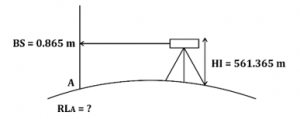# GATE’22 CE: Daily Practices Quiz. 16-July-2021

Quiz: Civil Engineering
Exam: GATE
Topic: Miscellaneous

Each question carries 2 marks
Negative marking: 1/3 mark
Time: 12 Minutes

Q1. The back sight at a station A is 0.865 m and the height of instrument is 561.365 m. the reduced level at A will be (in unit m)
(a) 558.705
(b) 559.260
(c) 560.500
(d) 560.550

Q2. A trapezoidal channel with base of 6 m and side slope of two horizontal to one vertical conveys water at 17 m³/sec with a depth of 1.5 m. The flow situation in the channel is:
(a) Critical
(b) Supercritical
(c) Subcritical
(d) None of the above

Q3. The area between the two isohyets 45 cm and 55 cm is 100 km², and that between 55 cm and 65 cm is 150 km². what is the average depth of annual precipitation over the basin of 250 km²?
(a) 50 cm
(b) 52 cm
(c) 56 cm
(d) 60 cm

Q4. The following data were obtained when a sample of medium sand was tested in a constant head permeameter:
Cross-section area of sample : 100 cm²
Discharge collected : 10 cc/s
The coefficient of permeability of the sand is
(a) 0.1 m/s
(b) 0.01 m/s
(c) 1 × 10^(-4) m/s
(d) 1 × 10^(-8) m/s

Q5. A crest vertical curve joins two gradients of +3% and -2% for a design speed of 80 km/h and the corresponding stopping sight distance of 120 m. the height of driver’s eye and the object above the road surface are 1.20 m and 0.15 m respectively. The curve length (which is less than stopping sight distance) to be provided is
(a) 120 m
(b) 152 m
(c) 163 m
(d) 240 m

Solutions

S1. Ans.(c)
Sol.HI=RL_A+BS
RL_A=HI-BS
RL_A=561.365-0.865
▭(RL_A=560.500 m.)

S2. Ans.(c)
Sol. Given,
B = 6m.
Q = 17 m³/s
y = 1.5 m.
2H:1V = mH:1V ⇒ m = 2
T = B + 2my
T = 6 + (2 × 2 × 1.5)
▭(T=12m)
A = (B + my) y
= [6 + (2 × 1.5)] × 1.5
= 13.5 m²
We know,
Froude no.(F)=(Q^2 T)/(gA^3 )
=((17)^2×12)/(9.81×(13.5)^3 )
▭(F=0.14368<1) Hence,flow is Subcritical

S3. Ans.(c)
Sol.
P_1=45 cm.
P_2=55 cm
P_3=65 cm
A_1=100 km^2
A_2=150 km^2
P_avg= ?
Average depth of annual precipitation by Iso-hyetal method is given by →
P_avg=(((P_1+P_2)/2).A_1+((P_2+P_3)/2) A_2)/(A_1+A_2 )
=(((45+55)/2)×100+((55+65)/2)×150)/(100+150)
=(5000+9000)/250
▭(P_avg=56 cm)

S4. Ans.(c)
Sol. Given,
Q = 10 cc/sec.
i = 10
A = 100 cm²
K = ?
We know,
Q = KiA
10 = K × 10 × 100
K=1/100 cm\/sec⁡
▭(K=1×10^(-4) m\/sec.)

S5. Ans.(b)
Sol. Given,
N_1= +3%=0.03
N_2= -2%= -0.02
N=|N_1-N_2 |=|0.03-(-0.02)|=0.05
S=120m
Note→ When, ▭(SSD<Curve length), then curve length is given by –
▭(L=2S-4.4/N)
=(2×120)-4.4/0.05
=240-88
▭(L=152m)

×Join India's largest learning destination

What You Will get ?

•Job Alerts
•Daily Quizzes
•Subject-Wise Quizzes
•Current Affairs
•Previous year question papers
•Doubt Solving session

ORJoin India's largest learning destination

What You Will get ?

•Job Alerts
•Daily Quizzes
•Subject-Wise Quizzes
•Current Affairs
•Previous year question papers
•Doubt Solving session

ORJoin India's largest learning destination

What You Will get ?

•Job Alerts
•Daily Quizzes
•Subject-Wise Quizzes
•Current Affairs
•Previous year question papers
•Doubt Solving session

Enter the email address associated with your account, and we'll email you an OTP to verify it's you.Join India's largest learning destination

What You Will get ?

•Job Alerts
•Daily Quizzes
•Subject-Wise Quizzes
•Current Affairs
•Previous year question papers
•Doubt Solving session

Enter OTP

Please enter the OTP sent to
/6

Did not recive OTP?

Resend in 60sJoin India's largest learning destination

What You Will get ?

•Job Alerts
•Daily Quizzes
•Subject-Wise Quizzes
•Current Affairs
•Previous year question papers
•Doubt Solving sessionJoin India's largest learning destination

What You Will get ?

•Job Alerts
•Daily Quizzes
•Subject-Wise Quizzes
•Current Affairs
•Previous year question papers
•Doubt Solving session

Almost there

+91Join India's largest learning destination

What You Will get ?

•Job Alerts
•Daily Quizzes
•Subject-Wise Quizzes
•Current Affairs
•Previous year question papers
•Doubt Solving session

Enter OTP

Please enter the OTP sent to Edit Number

Did not recive OTP?

Resend 60

By skipping this step you will not recieve any free content avalaible on adda247, also you will miss onto notification and job alerts

Are you sure you want to skip this step?

By skipping this step you will not recieve any free content avalaible on adda247, also you will miss onto notification and job alerts

Are you sure you want to skip this step?